# Application of Calculus (IB SL)

$\def\frac{\dfrac}$
1 (IB/sl/2019/May/paper1tz1/q10)
[Maximum mark: 17]
Consider $f(x)=\sqrt{x} \sin \left(\frac{\pi}{4} x\right)$ and $g(x)=\sqrt{x}$ for $x \geq 0$. The first time the graphs of $f$ and $g$ intersect is at $x=0$.
 (a) Find the two smallest non-zero values of $x$ for which $f(x)=g(x)$.

The set of all non-zero values that satisfy $f(x)=g(x)$ can be described as an arithmetic sequence, $u_{v}=a+b n$ where $n \geq 1$.
 (b) Find the value of $a$ and of $b$. $$
 (c) At point $\mathrm{P}$, the graphs of $f$ and $g$ intersect for the 21 st time. Find the coordinates of $\mathrm{P}$. $$

The following diagram shows part of the graph of $g$ reflected in the $x$-axis. It also shows part of the graph of $f$ and the point $\mathrm{P}$.
 (d) Find an expression for the area of the shaded region. Do not calculate the value of the expression.

2 (IB/sl/2019/May/paper1tz2/q10)
[Maximum mark: 14]
Let $y=\left(x^{3}+x\right)^{\frac{3}{2}}$
 (a) Find $\frac{\mathrm{d} y}{\mathrm{~d} x}$. 
 (b) Hence find $\int\left(3 x^{2}+1\right) \sqrt{x^{3}+x} d x$.

Consider the functions $f(x)=\sqrt{x^{3}+x}$ and $g(x)=6-3 x^{3} \sqrt{x^{3}+x}$, for $x \geq 0$.
The graphs of $f$ and $g$ are shown in the following diagram.
The shaded region $R$ is enclosed by the graphs of $f, g$, the $y$-axis and $x=1$.
 (c) Write down an expression for the area of $R$.
 (d) Hence find the exact area of $R$. 

3 (IB/sl/2019/May/paper2tz2/q2)
[Maximum mark: 5]
Let $f(x)=4-2 \mathrm{e}^{\top}$. The following diagram shows part of the graph of $f$.

 (a) Find the $x$-intercept of the graph of $f$.
 (b) The region enclosed by the graph of $f$, the $x$-axis and the $y$-axis is rotated $360^{\prime}$ about the $x$-axis. Find the volume of the solid formed.

 4 (IB/s1/2018/November/Paper1/q6) [Maximum mark: 8] Let $f(x)=\frac{6-2 x}{\sqrt{16+6 x-x^{2}}}$. The following diagram shows part of the graph of $f$.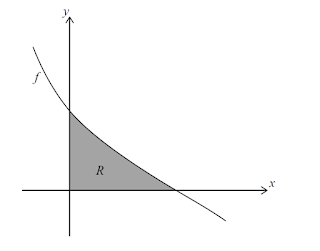The region $R$ is enclosed by the graph of $f$, the $x$-axis, and the $y$-axis. Find the area of $R$.

5 (IB/s1/2018/May/paper1tz1/q5)
[Maximum mark: 7]
Let $f(x)=\frac{1}{\sqrt{2 x-1}}$, for $x>\frac{1}{2}$
 (a) Find $\int(f(x))^{2} \mathrm{~d} x$.
 (b) Part of the graph of $f$ is shown in the following diagram.The shaded region $R$ is enclosed by the graph of $f$, the $x$-axis, and the lines $x=1$ and $x=9$. Find the volume of the solid formed when $R$ is revolved $360^{\circ}$ about the $x$-axis.

6 (IB/s1/2018/May/paper1tz2/q2)
[Maximum mark: 6]
Let $f(x)=6 x^{2}-3 x$. The graph of $f$ is shown in the following diagram.

 (a) Find $\int\left(6 x^{2}-3 x\right) d x$.
 (b) Find the area of the region enclosed by the graph of $f$, the $x$-axis and the lines, $x=1$ and $x=2$.

7 (IB/sl/2018/May/paper2tz2/q3)
[Maximum mark: 5]
Let $f(x)=\sin \left(\mathrm{e}^{t}\right)$ for $0 \leq x \leq 1.5$. The following diagram shows the graph of $f$.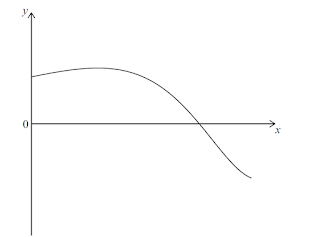(a) Find the $x$-intercept of the graph of $f$. 
 (b) The region enclosed by the graph of $f$, the $y$-axis and the $x$-axis is rotated $360^{\circ}$ about the $x$-axis. Find the volume of the solid formed. 

8 (IB/s1/2017/November/Paper2/q5)
[Maximum mark: 5]
Let $f(x)=6-\ln \left(x^{2}+2\right)$, for $x \in \mathbb{R}$. The graph of $f$ passes through the point $(p, 4)$. where $p>0$.
 (a) Find the value of $p$.
 (b) The following diagram shows part of the graph of $f$.

The region enclosed by the graph of $f$, the $x$-axis and the lines $x=-p$ and $x=p$ is rotated $360^{\circ}$ about the $x$-axis. Find the volume of the solid formed,

9 (IB/s1/2017/May/paper1tz2/q10)
[Maximum mark: 17]
Let $f(x)=x^{2}$. The following diagram shows part of the graph of $f$.

The line $L$ is the tangent to the graph of $f$ at the point $\mathrm{A}\left(-k, k^{2}\right)$, and intersects the $x$-axis at point B. The point C is $(-k, 0)$
 (a) (i) Write down $f^{\prime}(x)$ (ii) Find the gradient of $L$.
 (b) Show that the $x$-coordinate of $\mathrm{B}$ is $-\frac{k}{2}$,
 (c) Find the area of triangle $\mathrm{ABC}$, giving your answer in terms of $k$.

The region $R$ is enclosed by $L$, the graph of $f$, and the $x$-axis. This is shown in the following diagram.
 (d) Given that the area of triangle $\mathrm{ABC}$ is $p$ times the area of $R$, find the value of $p$, $$

10 (IB/sl/2017/May/paper2tz1/q10)
[Maximum mark: 15]
Let $f(x)=\ln x$ and $g(x)=3+\ln \left(\frac{x}{2}\right)$, for $x>0$ The graph of $g$ can be obtained from the graph of $f$ by two transformations: a horizontal stretch of scale factor $q$ followed by a translation of $\left(\begin{array}{l}h \\ k\end{array}\right)$.
 (a) (a) Write down the value of (i) $q$; (ii) $h$; (iii) $k$.

Let $h(x)=g(x) \times \cos (0.1 x)$, for $0 < x < 4$. The following diagram shows the graph of $h$ and the line $y=x$.

The graph of $h$ intersects the graph of $h^{-1}$ at two points. These points have $x$ coordinates $0.111$ and $3.31$, correct to three significant figures.
 (b) (i) Find $\int_{0.11}^{131}(h(x)-x) \mathrm{dx}$. (ii) Hence, find the area of the region enclosed by the graphs of $h$ and $h^{-1}$.
 (c) Let $d$ be the vertical distance from a point on the graph of $h$ to the line $y=x$. There is a point $\mathrm{P}(a, b)$ on the graph of $h$ where $d$ is a maximum. Find the coordinates of $\mathrm{P}$, where $0.111 < a < 3.31$.

11 (IB/sl/2016/November/Paper2/q6)
[Maximum mark: 6]
All lengths in this question are in metres.
Let $f(x)=-0.8 x^{2}+0.5$, for $-0.5 \leq x \leq 0.5$. Mark uses $f(x)$ as a model to create a barrel. The region enclosed by the graph of $f$, the $x$-axis, the line $x=-0.5$ and the line $x=0.5$ is rotated $360^{\circ}$ about the $x$-axis. This is shown in the following diagram.
 (a) Use the model to find the volume of the barrel.
 (b) The empty barrel is being filled with water. The volume $V \mathrm{~m}^{5}$ of water in the barrel after $t$ minutes is given by $V=0.8\left(1-\mathrm{e}^{-0.1 t}\right)$. How long will it take for the barrel to be half-full?

12 (IB/s1/2016/May/paper1tz2/q10)
[Maximum mark: 16]
The following diagram shows the graph of $f(x)=2 x \sqrt{a^{2}-x^{2}}$, for $-1 \leq x \leq a$, where $a>1$.

The line $L$ is the tangent to the graph of $f$ at the origin, $O$. The point $P(a, b)$ lies on $L$.
 (a) (i) Given that $f(x)=\frac{2 a^{2}-4 x^{2}}{\sqrt{a^{2}-x^{2}}}$, for $-1 \leq x < a$, find the equation of $L$. (ii) Hence or otherwise, find an expression for $b$ in terms of $a$,

The point $Q(a, 0)$ lies on the graph of $f .$ Let $R$ be the region enclosed by the graph of $f$ and the $x$-axis. This information is shown in the following diagram.

Let $A_{R}$ be the area of the region $R$.
 (b) Show that $A_{R}=\frac{2}{3} a^{3}$.
 (c) Let $A_{T}$ be the area of the triangle $\mathrm{OPQ}$, Given that $A_{\mathrm{T}}=k A_{2}$, find the value of $k$. $$

 13 (IB/s1/2019/May/paper1tz1/q5) [Maximum mark: 5] The derivative of a function $f$ is given by $f^{\prime}(x)=2 \mathrm{e}^{-3 x}$. The graph of $f$ passes through $\left(\frac{1}{3}, 5\right)$. Find $f(x)$.

14 (IB/s1/2017/May/paper1tz1/q5)
[Maximum mark: 7]
 (a) Find $\int x \mathrm{e}^{v^{2}-1} \mathrm{~d} x$. $$
 (b) Find $f(x)$. given that $f^{\prime}(x)=x \mathrm{e}^{2}-1$ and $f(-1)=3$.

 15 (IB/s1/2017/May/paper1tz2/q5) [Maximum mark: 6] Let $f^{\prime}(x)=\frac{3 x^{2}}{\left(x^{3}+1\right)^{5}}$, Given that $f(0)=1$, find $f(x)$.

 16 (IB/s1/2016/November/Paper1/q6) [Maximum mark: 7] Let $f^{\prime}(x)=\sin ^{3}(2 x) \cos (2 x)$. Find $f(x)$, given that $f\left(\frac{\pi}{4}\right)=1$.

17 (IB/s1/2019/November/Paper2/q10)
[Maximum mark: 14]
A rocket is travelling in a straight line, with an initial velocity of $140 \mathrm{~m} \mathrm{~s}^{-1}$. It accelerates to a new velocity of $500 \mathrm{~m} \mathrm{~s}^{-1}$ in two stages.
During the first stage its acceleration, $a \mathrm{~ms}^{-2}$, after $t$ seconds is given by $a(t)=240 \sin (2 t)$. where $0 \leq t \leq k$.
 (a) Find an expression for the velocity, $v \mathrm{~ms}^{-1}$, of the rocket during the first stage.

The first stage continues for $k$ seconds until the velocity of the rocket reaches $375 \mathrm{~ms}^{-1}$.
 (b) Find the distance that the rocket travels during the first stage.

During the second stage, the rocket accelerates at a constant rate. The distance which the rocket travels during the second stage is the same as the distance it travels during the first stage.
 (c) Find the total time taken for the two stages.

18 (IB/s1/2019/May/paper1tz1/q7)
[Maximum mark: 7]
A particle $\mathrm{P}$ starts from point $\mathrm{O}$ and moves along a straight line. The graph of its velocity, $\mathrm{vms}^{-11}$ after $t$ seconds, for $0 \leq t \leq 6$, is shown in the following diagram.

The graph of $v$ has $t$-intercepts when $t=0,2$ and 4 .
The function $s(t)$ represents the displacement of $\mathrm{P}$ from $\mathrm{O}$ after $t$ seconds.
It is known that $\mathrm{P}$ travels a distance of 15 metres in the first 2 seconds. It is also known that $s(2)=s(5)$ and $\int_{2}^{4} v \mathrm{~d} t=9$
 (a) Find the value of $s(4)-s(2)$. 
 (b) Find the total distance travelled in the first 5 seconds. 

19 (IB/s1/2019/May/paper2tz2/q8)
[Maximum mark: 16]
In this question distance is in centimetres and time is in seconds.
Particle $\mathrm{A}$ is moving along a straight line such that its displacement from a point $\mathrm{P}$, after $t$ seconds, is given by $s_{A}=15-t-6 t^{3} \mathrm{e}^{-0.1 .5 t}, 0 \leq t \leq 25$. This is shown in the following diagram.
 (a) Find the initial displacement of particle $\mathrm{A}$ from point $\mathrm{P}$. 
 (b) Find the value of $t$ when particle A first reaches point P. 
 (c) Find the value of $t$ when particle A first changes direction. 
 (d) Find the total distance travelled by particle $\mathrm{A}$ in the first 3 seconds. $$

Another particle, $\mathrm{B}$, moves along the same line, starting at the same time as particle $\mathrm{A}$. The velocity of particle $\mathrm{B}$ is given by $\mathrm{V}_{\mathrm{A}}=8-2 t, 0 \leq t \leq 25$.
 (e) (i) Given that particles $\mathrm{A}$ and $\mathrm{B}$ start at the same point, find the displacement function $s_{\mathrm{B}}$ for particle $\mathrm{B}$. (ii) Find the other value of $t$ when particles $\mathrm{A}$ and $\mathrm{B}$ meet. $$

20 (IB/s1/2018/November/Paper2/q4)
[Maximum mark: 7]
A particle moves along a straight line so that its velocity, $v \mathrm{~m} \mathrm{~s}^{-1}$, after $t$ seconds is given by $v(t)=1.4^{\prime}-2.7$, for $0 \leq t \leq 5$.
 (a) Find when the particle is at rest. 
 (b) Find the acceleration of the particle when $t=2$. 
 (c) Find the total distance travelled by the particle. 

21 (IB/s1/2018/May/paper2tz2/q9)
[Maximum mark: 15]
A particle P moves along a straight line. The velocity $v \mathrm{~ms}^{-1}$ of P after $t$ seconds is given by $v(t)=7 \cos t-5 t^{\text {tow }}$, for $0 \leq t \leq 7$.
The following diagram shows the graph of $v$.
 (a) Find the initial velocity of $\mathrm{P}$. $$
 (b) Find the maximum speed of $P$. $$
 (c) Write down the number of times that the acceleration of $\mathrm{P}$ is $0 \mathrm{~ms}^{-2}$. 
 (d) Find the acceleration of $\mathrm{P}$ when it changes direction. $$
 (e) Find the total distance travelled by $\mathrm{P}$. $$

22 (IB/s1/2017/November/Paper2/q9)
[Maximum mark: 14]
Note: In this question, distance is in metres and time is in seconds.
A particle P moves in a straight line for five seconds. Its acceleration at time $t$ is given by $a=3 \vec{r}-14 t+8$, for $0 \leq t \leq 5$,
 (a) Write down the values of $t$ when $a=0$.
 (b) Hence or othenvise, find all possible values of $t$ for which the velocity of $\mathrm{P}$ is decreasing.

When $t=0$, the velocity of $\mathrm{P}$ is $3 \mathrm{~ms}^{-1}$.
 (c) Find an expression for the velocity of $\mathrm{P}$ at time $t$.
 (d) Find the total distance travelled by $\mathrm{P}$ when its velocity is increasing. $$

 23 (IB/s1/2017/May/paper2tz2/q7) [Maximum mark: 6] Note: In this question, distance is in metres and time is in seconds. A particle moves along a horizontal line starting at a fixed point $\mathrm{A}$. The velocity $v$ of the particle, at time $t$, is given by $v(t)=\frac{2 t^{2}-4 t}{t^{2}-2 t+2}$, for $0 \leq t \leq 5$. The following diagram shows the graph of $v$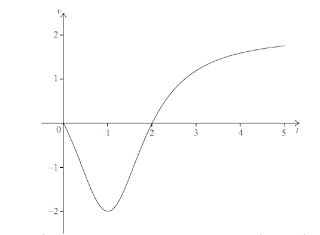There are $t$-intercepts at $(0,0)$ and $(2,0)$. Find the maximum distance of the particle from A during the time $0 \leq t \leq 5$ and justify your answer.

24 (IB/s1/2016/November/Paper2/q9)
[Maximum mark: 14]
A particle $\mathrm{P}$ starts from a point $\mathrm{A}$ and moves along a horizontal straight line,
Its velocity $v \mathrm{~cm} \mathrm{~s}^{-1}$ after $t$ seconds is given by
$$v(t)=\left\{\begin{array}{c}-2 t+2, \text { for } 0 \leq t \leq 1 \\3 \sqrt{t}+\frac{4}{t^{2}}-7, \text { for } 1 \leq t \leq 12 \end{array}\right.$$
The following diagram shows the graph of $v$.
 (a) Find the initial velocity of $\mathrm{P}$.

$\mathrm{P}$ is at rest when $t=1$ and $t=p$.
 (b) Find the value of $p$.

When $t=q$, the acceleration of $\mathrm{P}$ is zero.
 (c) (i) Find the value of $q$. (ii) Hence, find the speed of $\mathrm{P}$ when $t=q$.
 (d) (i) Find the total distance travelled by $\mathrm{P}$ between $t=1$ and $t=p$. (ii) Hence or othenvise, find the displacement of $\mathrm{P}$ from $\mathrm{A}$ when $\ell=p$.

 25 (IB/s1/2016/May/paper2tz2/q7) [Maximum mark: 7] A particle moves in a straight line. Its velocity $v \mathrm{~ms}^{-1}$ after $t$ seconds is given by $$v=6 t-6, \text { for } 0 \leq t \leq 2$$ After $p$ seconds, the particle is $2 \mathrm{~m}$ from its initial position. Find the possible values of $p$.

26 (IB/sl/2015/November/Paper1/q10)
[Maximum mark- 15]
Let $y=f(x)$, for $-0.5 \leq x \leq 6.5$. The following diagram shows the graph of $f^{\prime}$, the derivative of $f .$

The graph of $f^{\prime}$ has a local maximum when $x=2$, a local minimum when $x=4$, and it crosses the $x$-axis at the point $(5,0)$.
 (a) Explain why the graph of $f$ has a local minimum when $x=5$.
 (b) Find the set of values of $x$ for which the graph of $f$ is concave down. 

The following diagram shows the shaded regions $A, B$ and $C$.

The regions are enclosed by the graph of $f^{\prime}$, the $x$-axis, the $y$-axis, and the line $x=6$. The area of region $A$ is 12 , the area of region $B$ is $6.75$ and the area of region $C$ is $6.75$.
 (c) Given that $f(0)=14$, find $f(6)$.
 (d) Let $g(x)=(f(x))^{2}$. Given that $f^{\prime}(6)=16$, find the equation of the tangent to the graph of $g$ at the point where $x=6$.

 28 (IB/sl/2015/November/Paper1/q3) [Maximum mark: 6] Let $f^{\prime}(x)=6 x^{2}-5$. Given that $f(2)=-3$, find $f(x)$.

 29 (IB/s1/2015/May/paper1tz1/q7) [Maximum mark: 8] Let $f(x)=\cos x$, for $0 \leq x \leq 2 \pi$. The following diagram shows the graph of $f$. There are $x$-intercepts at $x=\frac{\pi}{2}, \frac{3 \pi}{2}$,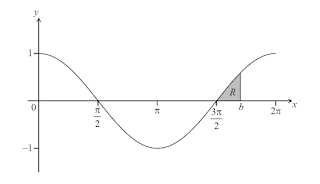The shaded region $R$ is enclosed by the graph of $f$, the line $x=b$, where $b>\frac{3 \pi}{2}$, and the $x$-axis. The area of $R$ is $\left(1-\frac{\sqrt{3}}{2}\right)$. Find the value of $b$.

30 (IB/s1/2015/May/paper1tz2/q10)
[Maximum mark: 14]
Consider a function $f$ with domain $-3 < x < 3$. The following diagram shows the graph of $f^{\prime}$, the derivative of $f$.

The graph of $f$ ' has $x$-intercepts at $x=a, x=0$, and $x=d$. There is a local maximum at $x=b$ and local minima at $x=a$ and at $x=c$.
 (a) Find all possible values of $x$ where the graph of $f$ is decreasing.
 (b) (i) Find the value of $x$ where the graph of $f$ has a local minimum. (ii) Justify your answer.
 (c) (c) The total area of the region enclosed by the graph of $f^{\prime}$ and the $x$-axis is 15 . Given that $f(a)=3$ and $f(d)=-1$, find the value of $f(0)$.

31 (IB/s1/2015/May/paper2tz1/q10)
[Maximum mark: 16]
Consider a function $f$, for $0 \leq x \leq 10 .$ The following diagram shows the graph of $f^{\prime}$. the derivative of $f$.

The graph of $f^{\prime}$ passes through $(2,-2)$ and $(5,1)$, and has $x$-intercepts at 0,4 and 6
 (a) The graph of $f$ has a local maximum point when $x=p$. State the value of $p$. and justify your answer.
 (b) Write down $f^{\prime}(2)$ $$

Let $g(x)=\ln (f(x))$ and $f(2)=3$
 (c) Find $g^{\prime}(2)$. 
 (d) Verify that $\ln 3+\int_{2}^{\alpha} g^{\prime}(x) \mathrm{d} x=g(a)$, where $0 \leq a \leq 10$ $$
 (e) The following diagram shows the graph of $g^{\prime}$, the derivative of $g$.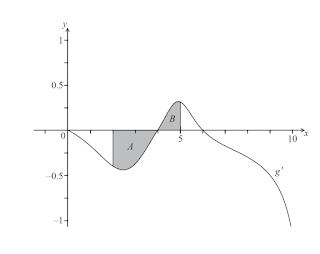The shaded region $A$ is enclosed by the curve, the $x$-axis and the line $x=2$, and has area $0.66$ units $^{2}$.
The shaded region $B$ is enclosed by the curve, the $x$-axis and the line $x=5$, and has area $0.21$ units $^{2}$.
Find $g(5)$ 

32 (IB/sl/2015/May/paper2tz1/q5)
[Maximum mark: 6]
Let $G(x)=95 \mathrm{e}^{-0.02 x}+40$, for $20 \leq x \leq 200$.
 (a) On the following grid, sketch the graph of $G$.

 (b) Robin and Pat are planning a wedding banquet. The cost per guest, $G$ dollars, is modelled by the function $G(n)=95 \mathrm{e}^{-1(0.0 v)}+40$, for $20 \leq n \leq 200$, where $n$ is the number of guests.

Calculate the total cost for 45 guests. 

33 (IB/s1/2015/November/Paper2/q6)
[Maximum mark: 6]
The velocity $v \mathrm{~m} \mathrm{~s}^{-1}$ of a particle after $t$ seconds is given by $$\mathrm{v}(t)=(0.3 t+0.1)^{\prime}-4, \text { for } 0 \leq t \leq 5$$
The following diagram shows the graph of $v$.
 (a) Find the value of $t$ when the particle is at rest. 
 (b) Find the value of $t$ when the acceleration of the particle is 0 . $$

(a) 2,10 (b) $a=-6,b=8$ (c) $(154,\sqrt{154})$ (d) $$\int_0^{158}\left(\sqrt{x}\sin\left(\frac{\pi}{4}\right)x+\sqrt{x}\right) dx$$

(a) $\frac{3}{2}\sqrt{x^3+x}(3x^2+1)$ (b) $\frac{2}{3}(x^3+x)^{\frac{3}{2}}$ (c) $\int_0^1(6-3x^2\sqrt{x^3+x}-\sqrt{x^3+x})-\sqrt{x^3+x}dx$ (d) 6-$\frac{4\sqrt{2}}{3}$

 (a) $x=\ln 2$ (b) volume $=3.43$

 area $=2$

 (a) $\frac{1}{2} \ln (2 x-1)+C$ (b) $\frac{\pi}{2} \ln 17$

 (a) $2 x^{3}-\frac{3 x^{2}}{2}+c$ (b) $\frac{19}{2}$

 (a) $x=\ln \pi$ (b) Volume $=2.50$

(a) $\quad p=\sqrt{e^{2}-2}$ (b) Volume $=332$

(a)(i) $f^{-1}(x)=2 x$ (ii) $-2 k$ (b) Show (c) $\frac{k^{3}}{4}$ (d) $p=3$

(a)(i) $q=2$ (ii) $h=0$ (iii) $k=3$ (b) (i) $2.72$ (ii) $5.45$ (c) $x=0.974, y=2.27$

(a) $V=0.601$ (b) $\quad t=4.71$

(a)(i) $y=2 a x-2 a^{2}+b$ (ii) $b=2 a^{2}$ (b) Show (c) $k=\frac{3}{2}$

 $y=-\frac{2}{3}e^{-3x}+5+\frac{2}{3}e^{-1}$

(a) $\frac{1}{2} e^{x^{2}-1}+c$ (b) $f(x)=\frac{1}{2} e^{x^{2}-1}+2.5$

 $f(x)=-\frac{1}{4}\left(x^{3}+1\right)^{-4}+\frac{5}{4}$

 $\quad f(x)=\frac{1}{8} \sin ^{4}(2 x)+\frac{7}{8}$

(a) $v=-120\cos(2t)+260$ (b) 354 (c) 2.23s

(a) 9 (b) 33

(a) 15 (b) $t=2.47$ (c) $t=4.08$ (d) $17.7(e)(i) S_{B}(t)=8 t-t^{2}+15$ (ii) $9.30$

(a) $\quad t=2.95$ (b) $a(2)=0.659$ (c) $5.35$

(a) $7$ (b) $24.7$ (c) 3 (d) $a=-9.25$ (e) $63.9$

(a) $t=\frac{2}{3}$ (b) $\frac{2}{3}<t<4$ (c) $v=t^{3}-7 t^{2}+8 t+3$ (d) $14.2$

 $2.28$

(a) $2$ (b) $p=5.22$ (c) (i) $q=1.95$ (ii) $1.76$ (d)(i) 4.45 (ii) $-3.45$

 $p=0.423$ or $p=1.58$

(a) $f^{\prime}(5)=0, f^{\prime \prime}(5)>0$ (b) $\quad 2<x<4$ (c) $f(6)=2$ (d) $y=64 x-380$

 $f(x)=2 x^{3}-5 x-9$

 $b=\frac{5 \pi}{3}$

(a) $0 \leqslant x \leqslant d$ (b) (i) $x=d$ (ii) sign change (c) $8.5$

(a) $p=6$ (b) $f^{\prime}(2)=-2$ (c) $g^{\prime}(2)=-\frac{2}{3}$ (d) Verify  (e) $g(5)=\ln 3-0.45$

(a) Graph (b) 3540

(a) $b=4.28$ (b) $t=1.19$